Related Articles
ML | Common Loss Functions
• Last Updated : 21 Nov, 2019

Loss function estimates how well particular algorithm models the provided data. Loss functions are classified into two classes based on the type of learning task –

• Regression Models : predict continuous values.
• Classification Models : predict the output from a set of finite categorical values.

REGRESSION LOSSES:

• Mean squared error
Also called as Quadratic Loss or L2 Loss.
It is the average of the squared difference between predictions and actual observations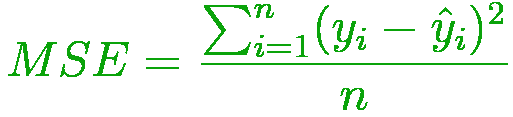```where,
i        - ith training sample in a dataset
n        - number of training samples
y(i)     - Actual output of ith training sample
y-hat(i) - Predicted value of ith traing sample```
• Mean Absolute error
Also known as L1 Loss.
It is the average of sum of absolute differences between predictions and actual observations.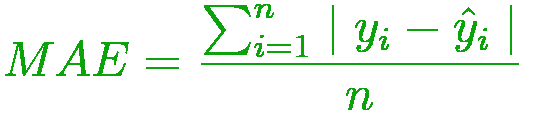• Mean Bias Error
same as MSE. It is less accurate but could conclude if the model has a positive bias or negative bias.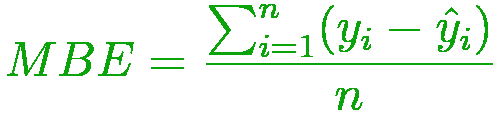• Huber Loss
also known as Smooth Mean Absolute Error. It is less sensitive to outliers in data than MSE and is also differentiable at 0. It is an absolute error, which becomes quadratic when the error is tiny.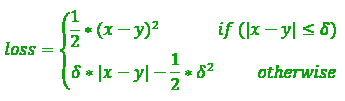CLASSIFICATION LOSSES:

• Cross Entropy Loss
also known as Negative Log Likelihood. It is the commonly used loss function for classification. Cross-entropy loss progress as the predicted probability diverges from actual label.• Hinge Loss
also known as Multi class SVM Loss. Hinge loss is applied for maximum-margin classification, prominently for support vector machines. It is a convex function used in convex optimizers.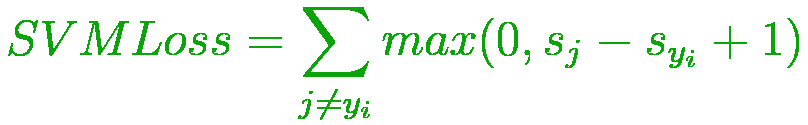My Personal Notes arrow_drop_up